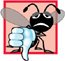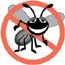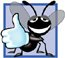# Example: Handling an Attempt to Divide by Zero

### Example Handling an Attempt to Divide by Zero

Let us consider a simple example of exception handling (Figs. 16.116.2). The purpose of this example is to prevent a common arithmetic problemdivision by zero. In C++, division by zero using integer arithmetic typically causes a program to terminate prematurely. In floating-point arithmetic, division by zero is allowedit results in positive or negative infinity, which is displayed as INF or -INF.

Figure 16.1. Class DivideByZeroException definition.

 ``` 1 // Fig. 16.1: DivideByZeroException.h 2 // Class DivideByZeroException definition. 3 #include // stdexcept header file contains runtime_error 4 using std::runtime_error; // standard C++ library class runtime_error 5 6 // DivideByZeroException objects should be thrown by functions 7 // upon detecting division-by-zero exceptions 8 class DivideByZeroException : public runtime_error 9 { 10 public: 11 // constructor specifies default error message 12 DivideByZeroException::DivideByZeroException() 13 : runtime_error( "attempted to divide by zero" ) {} 14 }; // end class DivideByZeroException ```

Figure 16.2. Exception-handling example that throws exceptions on attempts to divide by zero.

(This item is displayed on pages 814 - 815 in the print version)

``` 1 // Fig. 16.2: Fig16_02.cpp
2 // A simple exception-handling example that checks for
3 // divide-by-zero exceptions.
4 #include
5 using std::cin;
6 using std::cout;
7 using std::endl;
8
9 #include "DivideByZeroException.h" // DivideByZeroException class
10
11 // perform division and throw DivideByZeroException object if
12 // divide-by-zero exception occurs
13 double quotient( int numerator, int denominator )
14 {
15 // throw DivideByZeroException if trying to divide by zero
16 if ( denominator == 0 )
17 throw DivideByZeroException(); // terminate function
18
19 // return division result
20 return static_cast< double >( numerator ) / denominator;
21 } // end function quotient
22
23 int main()
24 {
25 int number1; // user-specified numerator
26 int number2; // user-specified denominator
27 double result; // result of division
28
29 cout << "Enter two integers (end-of-file to end): ";
30
31 // enable user to enter two integers to divide
32 while ( cin >> number1 >> number2 )
33 {
34 // try block contains code that might throw exception
35 // and code that should not execute if an exception occurs
36 try
37 {
38  result = quotient( number1, number2 );
39  cout << "The quotient is: " << result << endl;
40 } // end try
41
42 // exception handler handles a divide-by-zero exception
43 catch ( DivideByZeroException ÷ByZeroException )
44 {
45  cout << "Exception occurred: "
46  << divideByZeroException.what() << endl;
47 } // end catch
48
49 cout << "
Enter two integers (end-of-file to end): ";
50 } // end while
51
52 cout << endl;
53 return 0; // terminate normally
54 } // end main
```

 ``` Enter two integers (end-of-file to end): 100 7 The quotient is: 14.2857 Enter two integers (end-of-file to end): 100 0 Exception occurred: attempted to divide by zero Enter two integers (end-of-file to end): ^Z ```

In this example, we define a function named quotient that receives two integers input by the user and divides its first int parameter by its second int parameter. Before performing the division, the function casts the first int parameter's value to type double. Then, the second int parameter's value is promoted to type double for the calculation. So function quotient actually performs the division using two double values and returns a double result.

Although division by zero is allowed in floating-point arithmetic, for the purpose of this example, we treat any attempt to divide by zero as an error. Thus, function quotient tests its second parameter to ensure that it is not zero before allowing the division to proceed. If the second parameter is zero, the function uses an exception to indicate to the caller that a problem occurred. The caller (main in this example) can then process this exception and allow the user to type two new values before calling function quotient again. In this way, the program can continue to execute even after an improper value is entered, thus making the program more robust.

The example consists of two filesDivideByZeroException.h (Fig. 16.1) defines an exception class that represents the type of the problem that might occur in the example, and fig16_02.cpp (Fig. 16.2) defines the quotient function and the main function that calls it. Function main contains the code that demonstrates exception handling.

Defining an Exception Class to Represent the Type of Problem That Might Occur

Figure 16.1 defines class DivideByZeroException as a derived class of Standard Library class runtime_error (defined in header file ). Class runtime_errora derived class of Standard Library class exception (defined in header file )is the C++ standard base class for representing runtime errors. Class exception is the standard C++ base class for all exceptions. (Section 16.13 discusses class exception and its derived classes in detail.) A typical exception class that derives from the runtime_error class defines only a constructor (e.g., lines 1213) that passes an error-message string to the base-class runtime_error constructor. Every exception class that derives directly or indirectly from exception contains the virtual function what, which returns an exception object's error message. Note that you are not required to derive a custom exception class, such as DivideByZeroException, from the standard exception classes provided by C++. However, doing so allows programmers to use the virtual function what to obtain an appropriate error message. We use an object of this DivideByZeroException class in Fig. 16.2 to indicate when an attempt is made to divide by zero.

Demonstrating Exception Handling

The program in Fig. 16.2 uses exception handling to wrap code that might throw a "divide-by-zero" exception and to handle that exception, should one occur. The application enables the user to enter two integers, which are passed as arguments to function quotient (lines 1321). This function divides the first number (numerator) by the second number (denominator). Assuming that the user does not specify 0 as the denominator for the division, function quotient returns the division result. However, if the user inputs a 0 value as the denominator, function quotient tHRows an exception. In the sample output, the first two lines show a successful calculation, and the next two lines show a failed calculation due to an attempt to divide by zero. When the exception occurs, the program informs the user of the mistake and prompts the user to input two new integers. After we discuss the code, we will consider the user inputs and flow of program control that yield these outputs.

Enclosing Code in a TRy Block

The program begins by prompting the user to enter two integers. The integers are input in the condition of the while loop (line 32). After the user inputs values that represent the numerator and denominator, program control proceeds into the loop's body (lines 3350). Line 38 passes these values to function quotient (lines 1321), which either divides the integers and returns a result, or throws an exception (i.e., indicates that an error occurred) on an attempt to divide by zero. Exception handling is geared to situations in which the function that detects an error is unable to handle it.

C++ provides try blocks to enable exception handling. A try block consists of keyword try followed by braces ({}) that define a block of code in which exceptions might occur. The TRy block encloses statements that might cause exceptions and statements that should be skipped if an exception occurs.

Note that a TRy block (lines 3640) encloses the invocation of function quotient and the statement that displays the division result. In this example, because the invocation to function quotient (line 38) can throw an exception, we enclose this function invocation in a TRy block. Enclosing the output statement (line 39) in the try block ensures that the output will occur only if function quotient returns a result.

Software Engineering Observation 16.2Exceptions may surface through explicitly mentioned code in a try block, through calls to other functions and through deeply nested function calls initiated by code in a try block.

Defining a catch Handler to Process a DivideByZeroException

Exceptions are processed by catch handlers (also called exception handlers), which catch and handle exceptions. At least one catch handler (lines 4347) must immediately follow each try block. Each catch handler begins with the keyword catch and specifies in parentheses an exception parameter that represents the type of exception the catch handler can process (DivideByZeroException in this case). When an exception occurs in a try block, the catch handler that executes is the one whose type matches the type of the exception that occurred (i.e., the type in the catch block matches the thrown exception type exactly or is a base class of it). If an exception parameter includes an optional parameter name, the catch handler can use that parameter name to interact with a caught exception object in the body of the catch handler, which is delimited by braces ({ and }). A catch handler typically reports the error to the user, logs it to a file, terminates the program gracefully or tries an alternate strategy to accomplish the failed task. In this example, the catch handler simply reports that the user attempted to divide by zero. Then the program prompts the user to enter two new integer values.

Common Programming Error 16.1It is a syntax error to place code between a try block and its corresponding catch handlers.

Common Programming Error 16.2Each catch handler can have only a single parameterspecifying a comma-separated list of exception parameters is a syntax error.

Common Programming Error 16.3It is a logic error to catch the same type in two different catch handlers following a single try block.

Termination Model of Exception Handling

If an exception occurs as the result of a statement in a TRy block, the try block expires (i.e., terminates immediately). Next, the program searches for the first catch handler that can process the type of exception that occurred. The program locates the matching catch by comparing the thrown exception's type to each catch's exception-parameter type until the program finds a match. A match occurs if the types are identical or if the thrown exception's type is a derived class of the exception-parameter type. When a match occurs, the code contained within the matching catch handler executes. When a catch handler finishes processing by reaching its closing right brace (}), the exception is considered handled and the local variables defined within the catch handler (including the catch parameter) go out of scope. Program control does not return to the point at which the exception occurred (known as the throw point), because the try block has expired. Rather, control resumes with the first statement (line 49) after the last catch handler following the try block. This is known as the termination model of exception handling. [Note: Some languages use the resumption model of exception handling, in which, after an exception is handled, control resumes just after the throw point.] As with any other block of code, when a try block terminates, local variables defined in the block go out of scope.

Common Programming Error 16.4Logic errors can occur if you assume that after an exception is handled, control will return to the first statement after the throw point.

Error-Prevention Tip 16.2With exception handling, a program can continue executing (rather than terminating) after dealing with a problem. This helps ensure the kind of robust applications that contribute to what is called mission-critical computing or business-critical computing.

If the try block completes its execution successfully (i.e., no exceptions occur in the TRy block), then the program ignores the catch handlers and program control continues with the first statement after the last catch following that TRy block. If no exceptions occur in a try block, the program ignores the catch handler(s) for that block.

If an exception that occurs in a TRy block has no matching catch handler, or if an exception occurs in a statement that is not in a try block, the function that contains the statement terminates immediately, and the program attempts to locate an enclosing try block in the calling function. This process is called stack unwinding and is discussed in Section 16.8.

Flow of Program Control When the User Enters a Nonzero Denominator

Consider the flow of control when the user inputs the numerator 100 and the denominator 7 (i.e., the first two lines of output in Fig. 16.2). In line 16, function quotient determines that the denominator does not equal zero, so line 20 performs the division and returns the result (14.2857) to line 38 as a double (the static_cast< double > in line 20 ensures the proper return value type). Program control then continues sequentially from line 38, so line 39 displays the division result and line 40 is the end of the try block. Because the try block completed successfully and did not throw an exception, the program does not execute the statements contained in the catch handler (lines 4347), and control continues to line 49 (the first line of code after the catch handler), which prompts the user to enter two more integers.

Flow of Program Control When the User Enters a Denominator of Zero

Now let us consider a more interesting case in which the user inputs the numerator 100 and the denominator 0 (i.e., the third and fourth lines of output in Fig. 16.2). In line 16, quotient determines that the denominator equals zero, which indicates an attempt to divide by zero. Line 17 throws an exception, which we represent as an object of class DivideByZeroException (Fig. 16.1).

Note that, to throw an exception, line 17 uses keyword throw followed by an operand that represents the type of exception to throw. Normally, a throw statement specifies one operand. (In Section 16.5, we discuss how to use a throw statement that specifies no operands.) The operand of a throw can be of any type. If the operand is an object, we call it an exception objectin this example, the exception object is an object of type DivideByZeroException. However, a throw operand also can assume other values, such as the value of an expression (e.g., throw x > 5) or the value of an int (e.g., throw 5). The examples in this chapter focus exclusively on throwing exception objects.

Common Programming Error 16.5Use caution when throwing the result of a conditional expression (?:), because promotion rules could cause the value to be of a type different from the one expected. For example, when throwing an int or a double from the same conditional expression, the conditional expression converts the int to a double. However, the catch handler always catches the result as a double, rather than catching the result as a double when a double is thrown, and catching the result as an int when an int is thrown.

As part of throwing an exception, the tHRow operand is created and used to initialize the parameter in the catch handler, which we discuss momentarily. In this example, the tHRow statement in line 17 creates an object of class DivideByZeroException. When line 17 throws the exception, function quotient exits immediately. Therefore, line 17 throws the exception before function quotient can perform the division in line 20. This is a central characteristic of exception handling: A function should throw an exception before the error has an opportunity to occur.

Because we decided to enclose the invocation of function quotient (line 38) in a try block, program control enters the catch handler (lines 4347) that immediately follows the try block. This catch handler serves as the exception handler for the divide-by-zero exception. In general, when an exception is thrown within a try block, the exception is caught by a catch handler that specifies the type matching the thrown exception. In this program, the catch handler specifies that it catches DivideByZeroException objectsthis type matches the object type thrown in function quotient. Actually, the catch handler catches a reference to the DivideByZeroException object created by function quotient's tHRow statement (line 17).

Performance Tip 16.2Catching an exception object by reference eliminates the overhead of copying the object that represents the thrown exception.

Good Programming Practice 16.1Associating each type of runtime error with an appropriately named exception object improves program clarity.

The catch handler's body (lines 4546) prints the associated error message returned by calling function what of base-class runtime_error. This function returns the string that the DivideByZeroException constructor (lines 1213 in Fig. 16.1) passed to the runtime_error base-class constructor.C++ How to Program (5th Edition)
ISBN: 0131857576
EAN: 2147483647
Year: 2004
Pages: 627

Similar book on Amazon